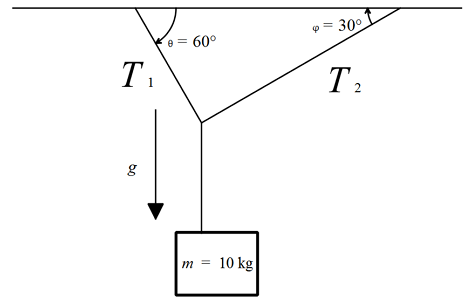# Slick TensionsAn object is hanging from a ceiling with the help of two cables arranged in such a way that a cable has a tension $T_1$ on the object and forms and angle $\theta=60^\circ$ with the ceiling and the other ones has a tension $T_2$ and makes an angle $\phi=30^\circ$. If the object has a mass $m=10 \text{ kg}$, calculate $|T_1| + |T_2|$ in Newtons to 2 decimal places.

In the image, $g$ is the acceleration due to gravity and has a value of $9.8 \text{ m/s}^2$.

×